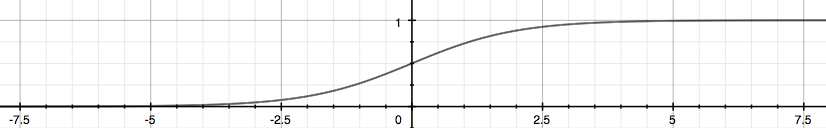×

Search anything:

# Logistic Regression using Scikit Learn

#### Machine Learning (ML) PythonGet this book -> Problems on Array: For Interviews and Competitive Programming

Reading time: 25 minutes | Coding time: 10 minutes

In this article, we will explore how to implement Logistic Regression in Python using Scikit Learn and create a real demo.

Logistic regression is a classification algorithm.So let's first discuss what is classification.

Classification
Unlike regression where we predict a continous value, we use classification to to predict a category. For example we can use classification algorithms to predict if the email is spam or not spam etc. Here in classification algorithms we predict a category.

Logistic regression
Logistic regression is a classification algorithm by which we can predict a category for given set.The sigmoid or logistic function looks like this:Now for binary classification(where there are ony two categories), the logistic regression model will return the category.

Scikit-learn
Scikit-learn is a maching learning library which has algorithms for linear regression, decision tree, logistic regression etc.

## Logistic regression in python using scikit-learn

Here is the code for logistic regression using scikit-learn

``````import numpy as np
import pandas as pd
import matplotlib.pyplot as plt
%matplotlib inline
``````

Importing the libraries numpy for linear algebra matrices, pandas for dataframe manipulation and matplotlib for plotting and we have written %matplotlib inline to view the plots in the jupyter notebook itself.

``````#importing the dataset
X=dataset.iloc[:,[2,3]].values
y=dataset.iloc[:,4].values
``````

Here we are importing the dataset Social_Network_Ads. It contains the data of people on a social network type the followin to get an insight of data

``````dataset.info()
``````

Here the X set contains two columns of:

• age
• salary of the people
• y contains the column of 0 or 1 which means the user purchsed the thing that the ads show or not.

Basiclly in this example we are trying to predict if the person on the social network sees an ad, then will he buy that product or not. 0 denotes he will not buy the product and 1 denotes that he will buy the product.

``````#splitting the dataset into train set and test set
from sklearn.model_selection import train_test_split
X_train,X_test,y_train,y_test=train_test_split(X,y, test_size=0.25,random_state=0)
``````

Here we are spitting the dataset into training set and test set.random_state is written to ensure that we get the same results.

``````#feature scaling
from sklearn.preprocessing import StandardScaler
sc_X=StandardScaler()
X_train=sc_X.fit_transform(X_train)
X_test=sc_X.transform(X_test)
``````

Feature scaling is done to ensure that we get all the features on the same scale. And to do that we import a class called StandardScaler.

``````#Fitting logistic regession model
from sklearn.linear_model import LogisticRegression
classifier=LogisticRegression(random_state=0)
classifier.fit(X_train,y_train)
``````

Here we are fitting our model. LogisticRegression is a class and classfier is an object of the LogisticRegression class.

``````#predicting the results
y_pred=classifier.predict(X_test)
``````

here we are predicting the results using the predict method.

Now we will evaluate our results using the confusion matrix

``````#evaluating the results
from sklearn.metrics import confusion_matrix
cm=confusion_matrix(y_test,y_pred)
cm
``````

you will get a matrix of:

``````[[65,  3],
[ 8, 24]]
``````

here the number of correct outputs or predictions is 65+24=89 and number of incorrect outputs is 8+3=11.

So we can conclude that our model is quite accurate.

## When to use logistic regression?

Logistic regression is used when we wnat to pedict a category or classify objects or things into categories.

Example:

• A customer will buy a product or not.
• An email is spam or not etc.